#### MAT_MM_CONCRETE

###### Material properties
Attention: This command is in the beta stage and the format may change over time.
*MAT_MM_CONCRETE
"Optional title"
mid, $\rho$, $G$
$K_0$, $K_L$, $cid_{cmp}$, $f_t$, $f_c$, $\xi$, $\lambda$, $\gamma$
$\xi_y$, $\xi_r$, $\varepsilon_{p,u0}$, $\varepsilon_{p,r0}$, $\psi_p$, $\psi_r$, $\varepsilon_{p,u}^{min}$, $\varepsilon_{p,r}^{min}$
$m$, $bulk$, $bulk_{cap}$, $cid_{src}$, $cid_{srt}$, $c$, $\sigma_{y,min}$, $\sigma_{y,max}$
$\mu$, $G_{r0}$, $L_{ref}$, $nsplit$
##### Parameter definition
VariableDescription
mid Unique material identification number
$\rho$ Density
$G$ Shear modulus
$K_0$ Bulk modulus of intact material
$K_L$ Bulk modulus at full compaction
$cid_{cmp}$ ID of CURVE with pressure vs. inelastic volumetric strain
$f_t$ Uniaxial tensile strength
$f_c$ Uniaxial compressive strength
$\xi$ Parameter controlling transition between region 2 to 3
default: 0.5
$\lambda$ Parameter controlling strength in region 2 and 3
default: 0.5
$\gamma$ Lode parameter dependency ( = 0 $\rightarrow$ Rankine, = 1 $\rightarrow$ von Mises)
$\xi_y$ Parameter controlling initial yield surface
$\xi_r$ Parameter controlling residual surface
$\varepsilon_{p,u0}$ Transition strain from initial yield surface to ultimate surface (at $p = f_c/3$)
$\varepsilon_{p,r0}$ Transition strain from ultimate surface to residual surface (at $p = f_c/3$)
$\psi_p$ Pressure dependency on transition strains
default: not used
$\psi_r$ Strain rate dependency on transition strains
default: not used
$\varepsilon_{p,u}^{min}$ Lower cap on $\varepsilon_{p,u}$
default: not used
$\varepsilon_{p,r}^{min}$ Lower cap on $\varepsilon_{p,r}$
default: not used
$m$ Exponent on damage factor
default: 1
$bulk$ Bulking parameter ranging between 0 and 1
default: not used
$bulk_{cap}$ Cap on volumetric strain caused by bulking
default: not used
$cid_{src}$ ID of CURVE with stress vs. plastic strain rate (at $p \ge f_c/3$)
$cid_{srt}$ ID of CURVE with stress vs. plastic strain rate (at $p \le -f_t/3$)
$c$ Viscous damping coefficient
default: not used
$\sigma_{y,min}$ Lower cap on flow stress
default: not used
$\sigma_{y,max}$ Upper cap on flow stress
default: not used
$\mu$ Deviation from nominal values
default: not used
$G_{r0}$ Energy per unit area in transition from ultimate to residual surface (at $p = f_c/3$)
default: not used
$L_{ref}$ Reference element length
default: not used
$nsplit$ Node splitting activation flag
options:
0 $\rightarrow$ node splitting inactive
1 $\rightarrow$ node splitting active
##### Description

Elastic and viscous stresses

The total stress, $\boldsymbol{\sigma}$, is the sum of an elastic component $\boldsymbol{\sigma^e}$ and a viscous component $\boldsymbol{\sigma^v}$.

$\boldsymbol{\sigma} = \boldsymbol{\sigma^e} + \boldsymbol{\sigma^v}$

Elastic strain component:

$\boldsymbol{\sigma^e} = 2\cdot G\boldsymbol{\varepsilon_{dev}^e} + K\cdot \boldsymbol{\varepsilon_{vol}^e}\cdot \mathbf{I}$

$G$ is the shear modulus, $\boldsymbol{\varepsilon_{dev}^e}$ is the elastic deviatoric strain, $K$ is the bulk modulus (defined below) and $\boldsymbol{\varepsilon_{vol}^e}$ is the elastic volumetric strain.

Viscous strain component:

$\boldsymbol{\sigma^v} = c\cdot \boldsymbol{\dot\varepsilon}$

$c$ is an input parameter and $\boldsymbol{\dot\varepsilon}$ is the total strain rate.

Inelastic compaction

Compaction is described by a curve of compaction pressure vs. densification (inelastic volumetric strain), defined by the user. From the defined curve, parameters $p_0$, $p_L$ and $\varepsilon_L$ are extracted by the solver. An example curve and the parameters extracted from it is presented below. The compaction pressure, $p_c\left(\varepsilon_{vol}^p\right)$, is increased from $p_0$ to $p_L$ during inelastic compaction, which occurs in region 3 (defined below).

Functions $f_u$ and $f_r$

Function $f_u\left(p,p_c,g\left(\theta\right)\right)$ is divided into three regions. Pressure, $p$, determines active region:

$\begin{array}{ccc} \mbox{Region} & \mbox{Pressure} & f_u \\ 1 & p \leq \frac{f_c}{3} & max\left(0, \eta\cdot \left(p-p_s\right)\right) \\ 2 & \frac{f_c}{3} \lt p \leq \xi\cdot p & f_u\left(\frac{f_c}{3},p_c,g\left(\theta\right)\right) + \lambda\cdot \eta\cdot \left(\xi\cdot p_c - f_c/3\right)\cdot \left(1 - \left(1 - \alpha\right)^{1/\lambda}\right) \\ 3 & p \gt \xi\cdot p & f_u\left(\xi\cdot p_c, p_c, g\left(\theta\right)\right) \end{array}$

where:

$\eta = \frac{g\left(\theta\right)\cdot f_c}{f_c/3-p_s}$
$p_s = \frac{\left(1+1/(2-\gamma)\right)\cdot f_t\cdot f_c}{3\cdot\left(f_t - f_c/(2-\gamma)\right)}$
$\alpha = \frac{p-f_c/3}{\xi\cdot p_c - f_c/3}$

$f_c$, $f_t$, $\xi$, $\lambda$ and $\gamma$ are input parameters and $g\left(\theta\right)$ is a function of Lode angle.

Function $f_r\left(p,p_c,g\left(\theta\right)\right)$ is defined as $f_r = max(0, f_u + \eta\cdot p_s)$. Functions $f_u$ and $f_r$ are used to describe the yield surface and the transition strains.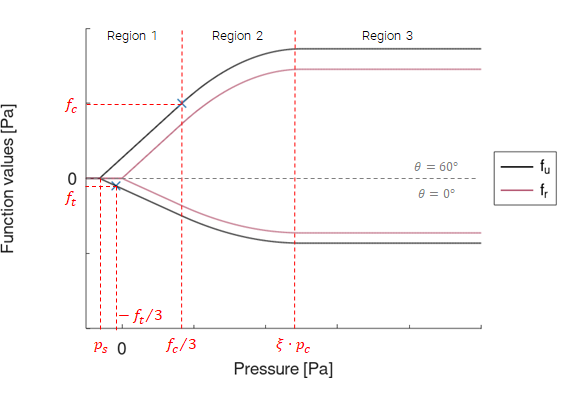Functions $f_u$ and $f_r$ in the case of uniaxial compression $\left(\theta = 60^{\circ}\right)$ and uniaxial tension $\left(\theta = 0^{\circ}\right)$ with parameter $\gamma = 0$ (Rankine). All values along the ordinate are positive.

Ultimate surface

The ultimate surface, $\sigma_u(p,p_c,g\left(\theta\right)$, is defined as function $f_u$ but with a cap in region 3:

$\displaystyle{\sigma_u = \left\{ \begin{array}{ccc} f_u & : & \mbox{Region 1 and 2} \\ f_u\left(\xi\cdot p_c,p_c,g\left(\theta\right)\right)\cdot \sqrt{1-min\left(1,\left(\frac{p-\xi\cdot p_c}{p_c\cdot (1-\xi)}\right)^2\right)} & : & \mbox{Region 3} \end{array} \right. }$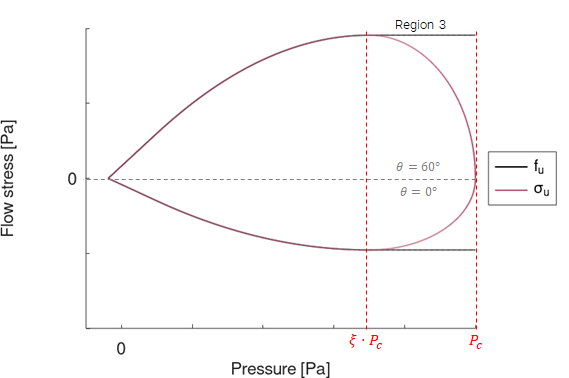Ultimate surface, $\sigma_u$ is defined as function $f_u$ but with a cap in region 3.

Parameters $f_c$, $f_t$, $\xi$, $\lambda$, $\gamma$ and $p_0$ control the shape of the ultimate surface: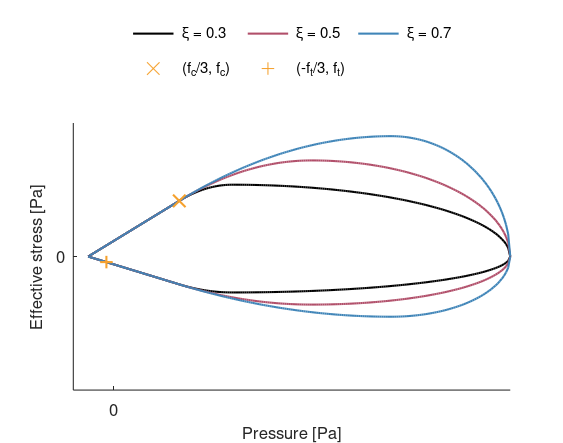Influence of parameter $\xi$. Transition between region 2 and 3 occurs at a higher pressure with increased value of $\xi$.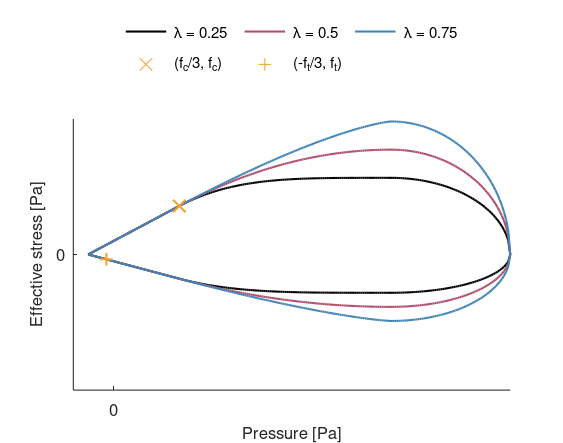Influence of parameter $\lambda$. Strength is increased in region 2 and 3 with increased value of $\lambda$.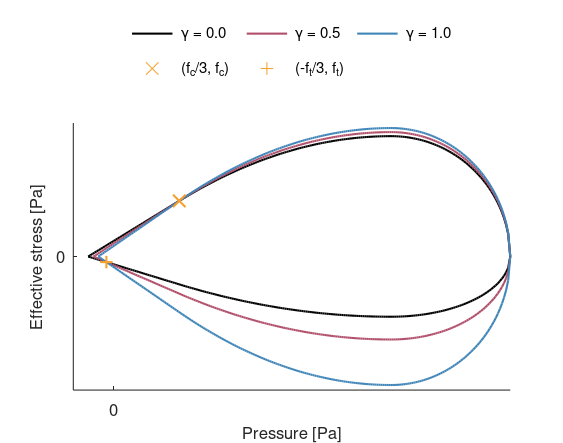Influence of parameter $\gamma$.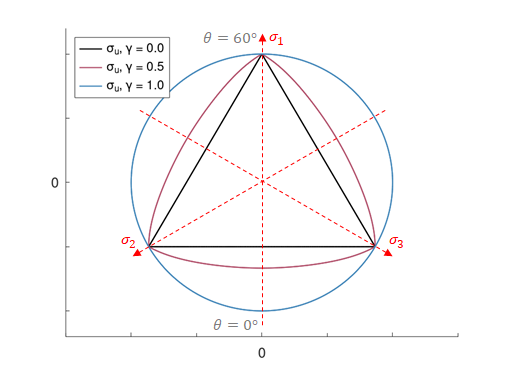Influence of parameter $\gamma$ displayed from $\pi$-plane. The surface is independent of Lode angle if $\gamma = 1$ (von Mises).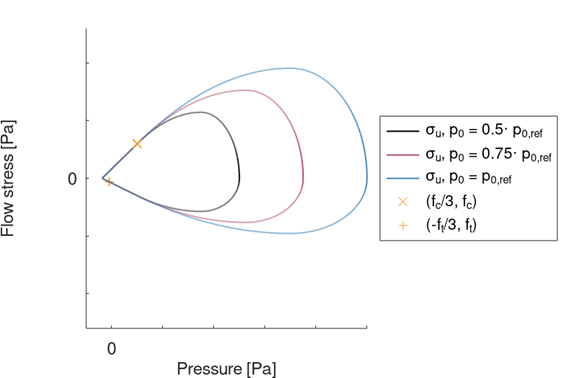Influence of parameter $p_0$.

In addition to demonstrating the influence of $p_0$, the figure above also demonstrates the evolution of the ultimate surface during inelastic compaction (from $p_c = 0.5\cdot p_{0,ref}$ to $p_c = p_{0,ref}$).

The maxium strength is increased with increased crushing pressure as:

$\sigma_u\left(\xi\cdot p_c, p_c, g\left(\theta\right)\right) = \sigma_u\left(\xi\cdot p_0, p_0, g\left(\theta\right)\right)\cdot \frac{\xi\cdot p_c - f_c/3}{\xi\cdot p_0 -f_c/3}$

Initial yield surface

The initial yield surface, $\sigma_{y0}\left(p,p_c,g\left(\theta\right)\right)$, is defined as:

$\sigma_{y0} = \xi_y\cdot \sigma_u$

$\xi_y$ is an input parameter.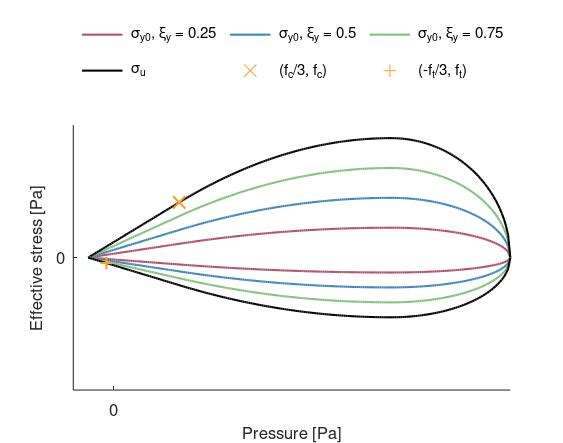Influence of parameter $\xi_y$.

Residual surface

The residual surface, $\sigma_r\left(p,p_c,g\left(\theta\right)\right)$, is defined as:

$\displaystyle{\sigma_r = \left\{ \begin{array}{ccc} \beta\cdot f_r & : & \mbox{Region 1 and 2} \\ \beta\cdot f_r\left(\xi\cdot p_c,p_c,g\left(\theta\right)\right)\cdot \sqrt{1-min\left(1,\left(\frac{p-\xi\cdot p_c}{p_c\cdot (1-\xi)}\right)^2\right)} & : & \mbox{Region 3} \end{array} \right. }$
$\beta = min\left(1, \xi_r\cdot \eta\cdot \frac{p-p_s}{f_u}\right)$

$\xi_r$ is an input parameter.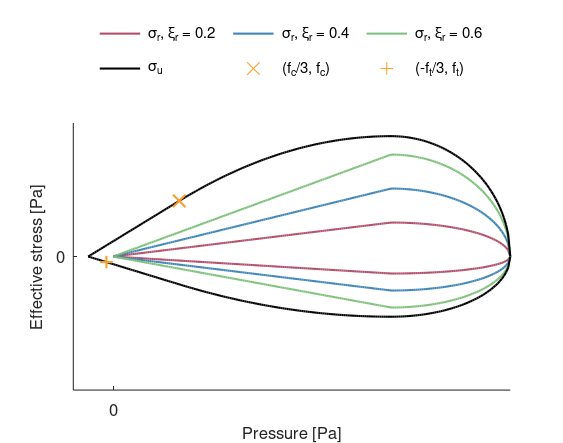Influence of parameter $\xi_r$.

Yield criterion and plastic flow

$\begin{array}{ccc} \sigma_{eff} = \sigma_y \rightarrow \mbox{Yielding} & : & \mbox{Region 1 and 2} \\ \left(\frac{\sigma_{eff}}{\sigma_y}\right)^2 + \left(\frac{p/p_c-\xi}{1-\xi}\right)^2 \rightarrow \mbox{Yielding} & : & \mbox{Region 3} \end{array}$

Parameter $bulk$ controls the type of plastic flow in region 1 and 2. With $bulk = 0$, the plastic flow is purely deviatoric. With $bulk = 1$, associated flow is used, meaning that the plastic flow is both deviatoric and volumetric. The volumetric strain caused by bulking can be capped with parameter $bulk_{cap}$. Radial return is used in region 3.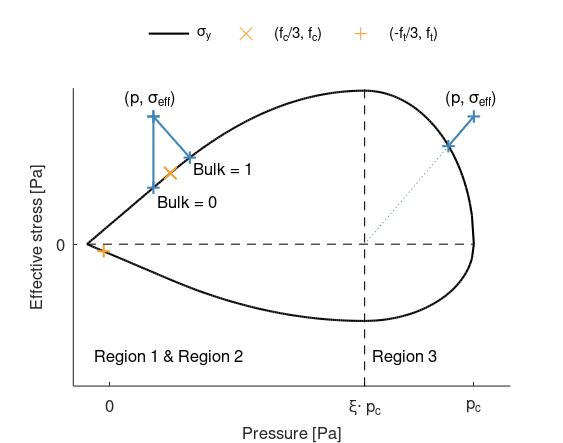Parameter $bulk$ controls type of plastic flow in region 1 and 2.

Damage

Damage is divided into tensile damage, $D_t$ and crushing damage, $D_c$. Crushing damage is divided into deviatoric crushing damage, $D_{c,dev}$, and volumetric crushing damage, $D_{c,vol}$.

Pressure and region determines which type of damage that accumlates. Damage grows with plastic flow and is initiated once the ultimate surface is reached. Node splitting, $nsplit$, is optional and is only used for tensile damage.

Tensile damage developes during negative pressures and does not affect the materials behavior in compression $\left(p \ge 0 \right)$.

$D_t = min\left(1,\sum{\frac{d\varepsilon_{eff}^p}{\varepsilon_r}}\right)$

Crushing damage develops during positive pressures and affects the materials behavior in tension $\left(p \lt 0 \right)$.

$D_c = \sqrt{D_{c,dev}^2 + D_{c,vol}^2}$
$D_{c,dev} = min\left(1,\sum{\frac{d\varepsilon_{eff}^p}{\varepsilon_r}}\right)$
$D_{c,vol} = min\left(1,\sum{\frac{d\varepsilon_{vol}^p}{\varepsilon_{L}}}\right)$

A damage factor, $D_{fac}$ is defined as:

$\displaystyle{D_{fac} = \left\{ \begin{array}{ccc} \left(1-D_c\right)^m & : & p \ge 0 \\ \left(1-D_c\right)^m\cdot \left(1-D_t\right)^m & : & p \lt 0 \end{array} \right. }$

$m$ is an input parameter.

Contour plot attribute "Damage" in the GUI displays maximum of tensile and crushing damage.

Strain rate dependency

Strain rate dependency is defined by curves containing stress vs. plastic strain rate. Parameters $cid_{src}$ and $cid_{srt}$ can refer to the same curve. In the case of different curves, the strain rate term, $\sigma_y^{rate}\left(sr,p\right)$, is pressure dependent and the following holds:

$\begin{array}{ccc} \mbox{Pressure} & & \sigma_y^{rate} \mbox{ defined by}\\ p \leq -\frac{f_t}{3} & : & \mbox{curve with id.$cid_{srt}$} \\ -\frac{f_t}{3} \lt p \leq \frac{f_c}{3} & : & \mbox{interpolation} \\ p \gt \frac{f_c}{3} & : & \mbox{curve with id.$cid_{src}$} \end{array}$

Parameter $sr$ is the plastic strain rate, defined as:

$\displaystyle{sr = \left\{ \begin{array}{ccc} \dot{\varepsilon}_{eff}^p & : & \mbox{Region 1 and 2} \\ \sqrt{\left(\dot{\varepsilon}_{eff}^p\right)^2 + \left(\dot{\varepsilon}_{vol}^p\right)^2} & : & \mbox{Region 3} \end{array} \right. }$

The strain rate term is added to the quasi-static yield stress and crushing pressure:

$\sigma_y^{dyn} = \sigma_y^{qs} + \sigma_y^{rate}$
$p_c^{dyn} = p_c^{qs} + \sigma_y^{rate}$

Transition strains

Transition from initial yield surface to ultimate surface and from ultimate surface to residual surface is controlled by parameters $\varepsilon_{p,u}\left(p,g\left(\theta\right),sr\right)$ and $\varepsilon_{p,r}\left(p,g\left(\theta\right),sr\right)$, respectively.

$\varepsilon_{p,u} = \varepsilon_{p,u0}\cdot\left(1 + \psi_p\cdot \left(\frac{f_u^*+\psi_r\cdot\sigma_y^{rate}}{f_c}-1\right)\right)$
$\varepsilon_{p,r} = \varepsilon_{p,r0}\cdot\left(1 + \psi_p\cdot \left(\frac{f_u^*+\psi_r\cdot\sigma_y^{rate}}{f_c}-1\right)\right)$

$\varepsilon_{p,r0}$, $\varepsilon_{p,u0}$, $\psi_r$ and $\psi_p$ are input parameters. Note that strain rate dependency requires $\psi_p\gt 0$ and $\psi_r\gt 0.$

Function $f_u^*$ is defined as function $f_u$ but independent of inelastic compaction, i.e. $f_u^*\left(p,g\left(\theta\right)\right) = f_u\left(p,p_0,g\left(\theta\right)\right)$.

An energy-based transition from ultimate to residual surface can be defined with parameter $G_{r0}$. The transition strain for negative pressures is then defined as:

$\varepsilon_{p,r}\left(p,g\left(\theta\right),sr,V_e\right) = \frac{G_{r0}}{V_e^{1/3}\cdot f_c}\cdot \left(1 + \psi_p\cdot \left(\frac{f_u^*+\psi_r\cdot\sigma_y^{rate}}{f_c}-1\right)\right)$

$V_e$ is the element volume, automatically set for each element at initialization. Note that $\varepsilon_{p,r0}$ still must be defined, since $G_{r0}$ only operates at negative pressures.

If parameter $L_{ref}$ is defined, parameter $\varepsilon_{p,r}^{min}$ is scaled with element size by a factor $L_{ref}/V_e^{1/3}$.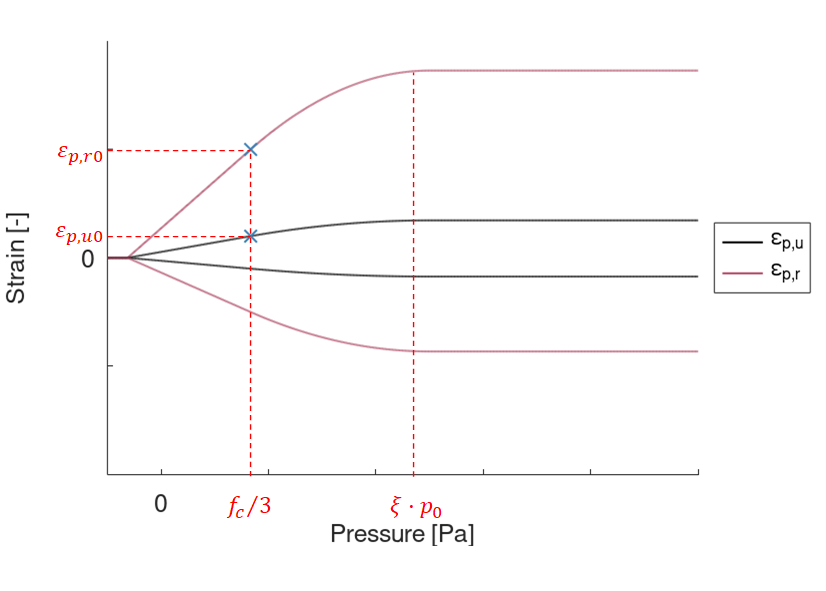The transition strains reach maximum at $p = \xi\cdot p_0$ and are constant for $p > \xi\cdot p_0$. Note that $\varepsilon_{p,u0}$ and $\varepsilon_{p,r0}$ are the values at $p = f_c/3$. Parameter $\psi_p = 1$ in the figure.

Flow stress

The flow stress, $\sigma_y\left(p,p_c,g\left(\theta\right),\varepsilon_{eff}^p,sr,D_{fac}\right)$, is defined as:

$\displaystyle{\sigma_y = \left\{ \begin{array}{ccc} \sigma_{y0} & : & \varepsilon_p^{eff} = 0 \mbox{ and } D_{fac} = 1 \\ \sigma_u\cdot h & : & \varepsilon_p^{eff} \gt 0 \mbox{ and } D_{fac} = 1 \\ \sigma_u\cdot D_{fac} + \sigma_r\left(1-D_{fac}\right) & : & \varepsilon_p^{eff} \gt 0 \mbox{ and } D_{fac} \lt 1 \end{array} \right. }$

The plastic hardening, $h$, is defined as:

$h = min\left(1,\sum{\frac{d\varepsilon_{eff}^p}{\varepsilon_u}}\right), h(0) = \xi_y$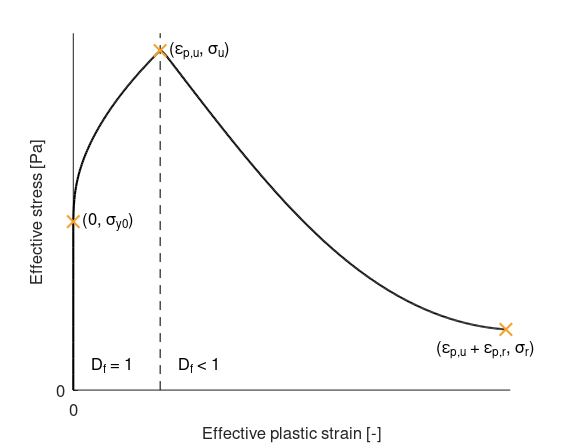Effective stress vs effective plastic from a compression test in region 2, illustrating the transition between the surfaces.

Caps on the flow stress can be defined with input parameters $\sigma_{y,max}$ and $\sigma_{y,min}$.

Modeling of material inhomogeneity

Parameter, $\mu$, introduces deviations to the surfaces and transition strains.

$rnd_f^1 = 1 + \mu\cdot\left(2\cdot rnd - 1\right)$
$rnd_f^2 = 1 - \mu\cdot\left(2\cdot rnd - 1\right)$

$rnd$ is a random number in the range [0,1]. Parameters $p_0$, $p_L$, $f_t$, $f_c$ are scaled with $rnd_f^1$ and parameter $\varepsilon_L$ with $rnd_f^2$. The scaling is done on integration point level at initialization.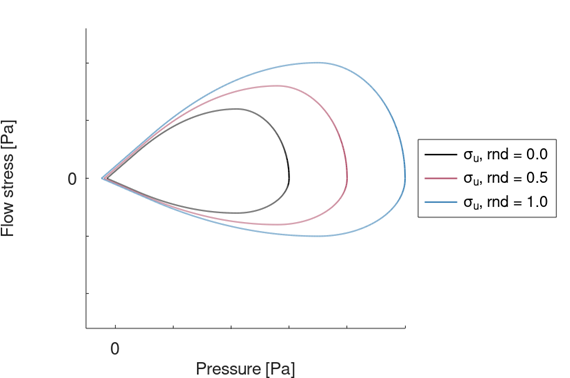Ultimate surface for three different integration points, demonstrating the effect of deviations $(\mu = 0.25)$ on $p_0$, $f_c$ and $f_t$. The surface with $rnd = 0.5$ corresponds to the surface based on nominal values of $p_0$, $p_L$, $f_t$ and $f_c$.